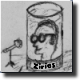MhdBanat -3 years ago 116
C Question

# Masks bits of array members

Can you direct me where is my problem here? I am trying to test values of an array.

Every value presents 16 bits (flags) and I need to check if the flag is set or not.

My current output is shown below and also the expected output.

`````` #include <stdio.h>

unsigned short value;
unsigned int bitCheck(unsigned int mask, int pin);

int main(void){
value=0;
value=4095;

int pin0 = 0;
int pin1 = 1;

unsigned int bit0= bitCheck( mask, pin0);
unsigned int bit1= bitCheck( mask, pin1);

for (int i =0;i<=1; i++)
{

if ( bit0 == 1 ){
printf("Pin %d is Set\n", pin0);
}else{
printf("Pin %d is not Set\n", pin0);
}

if ( bit1 == 1 ){
printf("Pin %d is Set\n", pin1);
}else{
printf("Pin %d is not Set\n", pin1);
}

printf("\n");
}

}

unsigned int bitCheck(unsigned int mask, int bit){
if ( (mask >> bit ) & 1){
return 1;
}else{
return 0;
}
}
``````

My output is:

``````Mask = 0 ==>>
Pin 0 is not Set
Pin 1 is not Set

Pin 0 is not Set
Pin 1 is not Set
``````

and it must be:

``````Mask = 0 ==>>
Pin 0 is not Set
Pin 1 is not Set

Pin 0 is Set
Pin 1 is Set
``````Felix Palmen

This code is unnecessarily complex and through it's complexity, hides a blatant logical error. You're doing your calls to `bitCheck(mask, ...)` before you ever assign a value to `mask`.

Change this:

``````    unsigned int bit0= bitCheck( mask, pin0);
unsigned int bit1= bitCheck( mask, pin1);

for (int i =0;i<=1; i++)
{
``````

to this:

``````  for (int i =0;i<=1; i++)
{

unsigned int bit0= bitCheck( mask, pin0);
unsigned int bit1= bitCheck( mask, pin1);

``````

That said, here's a sane way to do all this:

``````#include <stdio.h>

#define BIT(n, v) (!!((v) & (1U << (n))))

int main(void)
{
unsigned short values[] = {0, 4095};
for (int i = 0; i < 2; ++i)
{
printf("value: %hu\n", values[i]);
for (int bit = 0; bit < 16; ++bit)
{
printf( BIT(bit, values[i]) ?
"bit %d is set\n" :
"bit %d is not set\n", bit);
}
}
return 0;
}
``````

For one reason why your approach isn't considered sane, read about the DRY principle. Writing 16 times the same code is a simple example of violating this principle -> you need a loop instead.

Recommended from our users: Dynamic Network Monitoring from WhatsUp Gold from IPSwitch. Free Download